# NCERT Solutions for Class 9 Maths Exercise 4.1## myCBSEguide App

CBSE, NCERT, JEE Main, NEET-UG, NDA, Exam Papers, Question Bank, NCERT Solutions, Exemplars, Revision Notes, Free Videos, MCQ Tests & more.

NCERT solutions for Class 9 Maths Linear Equations in Two Variables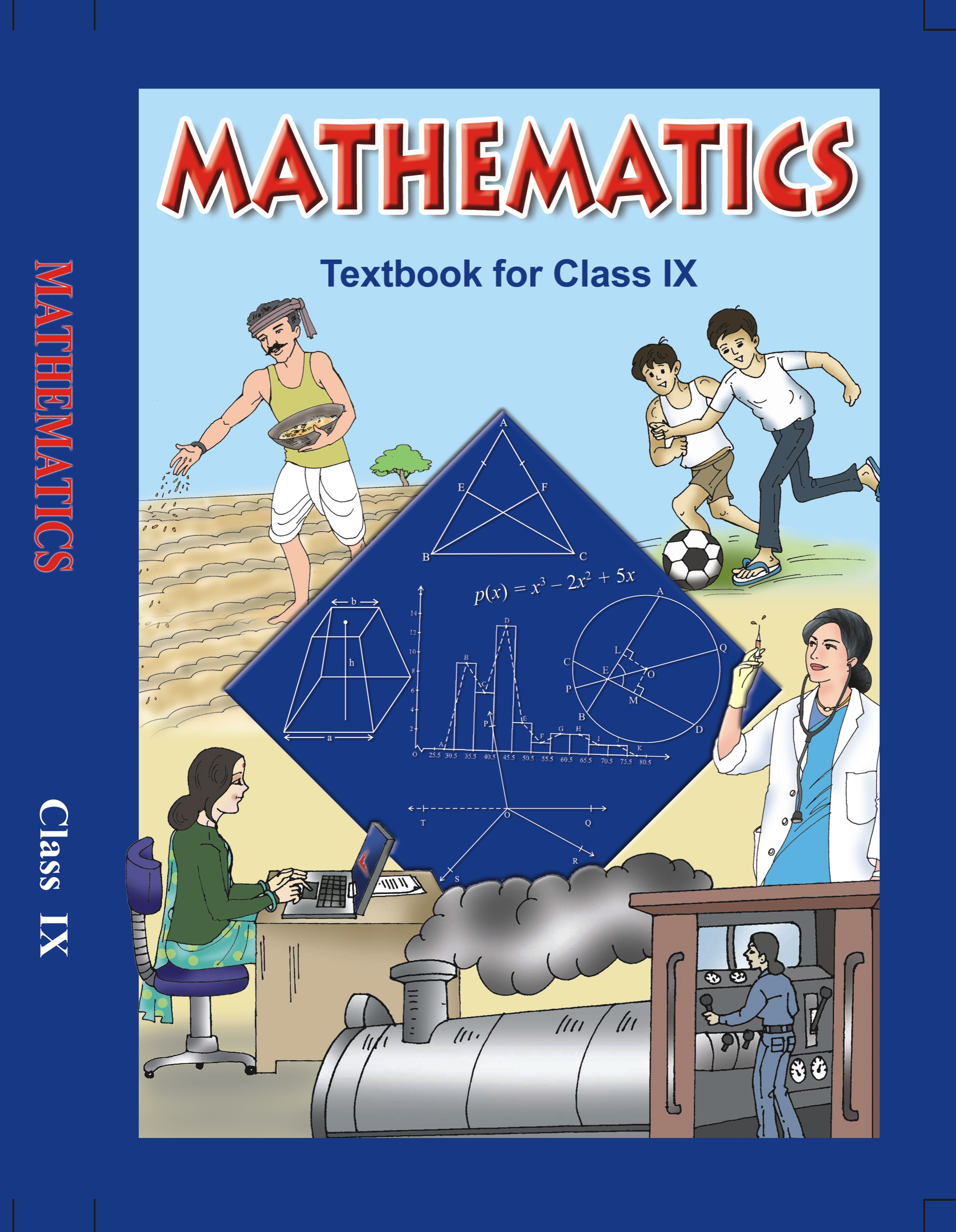## NCERT Solutions for Class 9 Mathematics Linear Equations in Two Variables

###### (Take the cost of a notebook to be Rs x and that of a pen to be Rs y).

Ans. Let the cost of a notebook be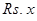.

Let the cost of a pen be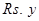.

We need to write a linear equation in two variables to represent the statement, “Cost of a notebook is twice the cost of a pen”.

Therefore, we can conclude that the required statement will be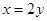.

NCERT Solutions for Class 9 Maths Exercise 4.1

###### 2. Express the following linear equations in the form ax + by + c = 0 and indicate the values of a, b and c in each case:

(i)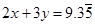(ii)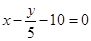(iii)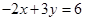(iv)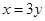(v)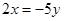(vi)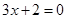(vii)(viii)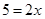Ans. (i)We need to express the linear equationin the form ax + by + c = 0 and indicate the values of a, b and c.can also be written as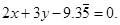We need to compare the equation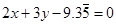with the general equation ax + by + c = 0, to get the values of a, b and c.

Therefore, we can conclude that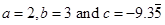.

###### (ii)We need to express the linear equationin the form ax + by + c = 0 and indicate the values of a, b and c.can also be written as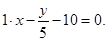We need to compare the equation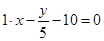with the general equation ax + by + c = 0, to get the values of a, b and c.

Therefore, we can conclude that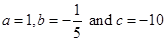.

###### (iii)We need to express the linear equationin the form ax + by + c = 0 and indicate the values of a, b and c.can also be written as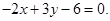We need to compare the equation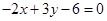with the general equation ax + by + c = 0, to get the values of a, b and c.

Therefore, we can conclude that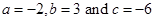.

###### (iv)We need to express the linear equationin the form ax + by + c = 0 and indicate the values of a, b and c.can also be written as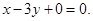We need to compare the equation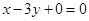with the general equation ax + by + c = 0, to get the values of a, b and c.

Therefore, we can conclude that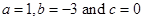.

###### (v)We need to express the linear equationin the form ax + by + c = 0 and indicate the values of a, b and c.can also be written as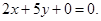We need to compare the equation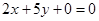with the general equation ax + by + c = 0, to get the values of a, b and c.

Therefore, we can conclude that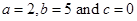.

###### (vi)We need to express the linear equationin the form ax + by + c = 0 and indicate the values of a, b and c.can also be written as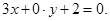We need to compare the equation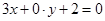with the general equation ax + by + c = 0, to get the values of a, b and c.

Therefore, we can conclude that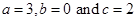.

NCERT Solutions for Class 9 Maths Exercise 4.1

###### (vii)We need to express the linear equationin the form ax + by + c = 0 and indicate the values of a, b and c.can also be written as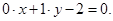We need to compare the equation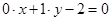with the general equation ax + by + c = 0, to get the values of a, b and c.

Therefore, we can conclude that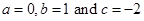.

(viii)We need to express the linear equationin the form ax + by + c = 0 and indicate the values of a, b and c.can also be written as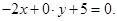We need to compare the equation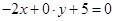with the general equation ax + by + c = 0, to get the values of a, b and c.

Therefore, we can conclude that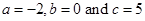.

## NCERT Solutions for Class 9 Maths Exercise 4.1

NCERT Solutions for Class 9 Maths PDF (Download) Free from myCBSEguide app and myCBSEguide website. Ncert solution class 9 Maths includes text book solutions from Mathematics Book. NCERT Solutions for CBSE Class 9 Maths have total 15 chapters. 9 Maths NCERT Solutions in PDF for free Download on our website. Ncert Maths class 9 solutions PDF and Maths ncert class 9 PDF solutions with latest modifications and as per the latest CBSE syllabus are only available in myCBSEguide.

## CBSE app for Class 9

To download NCERT Solutions for Class 9 Maths, Computer Science, Home Science,Hindi ,English, Social Science do check myCBSEguide app or website. myCBSEguide provides sample papers with solution, test papers for chapter-wise practice, NCERT solutions, NCERT Exemplar solutions, quick revision notes for ready reference, CBSE guess papers and CBSE important question papers. Sample Paper all are made available through the best app for CBSE students and myCBSEguide website.### 2 thoughts on “NCERT Solutions for Class 9 Maths Exercise 4.1”

1. It helped me a lot

2. Thanks for helping me